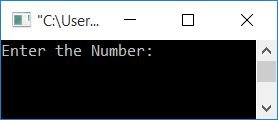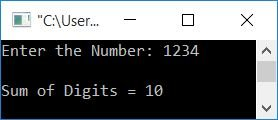# C++ program to find the sum of the digits of a number

In this article, you will learn and get code for finding the sum of digits of a given number given by the user at run-time using C++ programming. The same program is created with the following approaches:

To add digits to any number in C++ programming, you have to ask the user to enter the number to add its digits and display the addition result on the output screen, as shown here in the following program.

## Using the while loop, find the sum of a number's digits

Let's first start with the "while" loop. That is, the program given below finds and prints the sum of digits of a number using a while loop in C++.

```#include<iostream>
using namespace std;
int main()
{
int num, rem, sum=0;
cout<<"Enter the Number: ";
cin>>num;
while(num>0)
{
rem = num%10;
sum = sum+rem;
num = num/10;
}
cout<<"\nSum of Digits = "<<sum;
cout<<endl;
return 0;
}```

This program was built and runs under the Code::Blocks IDE. Here is its sample run:Now supply any number as input, say 1234, and press the ENTER key to see the summation result of its digit. The sum of digits of a number 1234 evaluates to 1+2+3+4 or 10, as shown in the output given below:The dry run of the above program goes like this:

• After receiving the number, say 1234, the variable num=1234.
• Because it is greater than 0, the condition evaluates to be true, and program flow goes inside the while loop.
• The value of num%10, 1234%10, or 4 is set to rem. So rem=4
• sum+rem or 0+4 (the sum's initial value is 0) or 4 is configured to sum
• The new sum value is 4.
• num/10 or 1234/10 or 123 is set to num.
• Again, it is greater than 0, therefore the condition of the while loop evaluates to be true, and again, the program flow goes inside the loop.
• The value of num%10, 123%10, or 3 is set to rem. So rem=3
• sum+rem or 4+3 or 7 is set to sum.
• The new value of sum is now 7.
• The value of num/10, 123/10, or 12 is set to num.
• Because it is greater than 0, the program flow goes inside the loop, and executes all three statements.
• Continue the operation until the value of num is equal to zero.
• After exiting the loop, we'll have a variable sum that holds the sum of the digits of the given number.
• As a result, simply print the sum value as output.

## Using the for loop, calculate the sum of a number's digits

The same program is created here using the for loop. Unlike the while loop, the initialization and update parts can also be written under the for loop. Therefore, we have initialized the value of sum=0 as the first statement of the for loop; the second statement is condition checking as done in the while loop; and at last, the update part is written as the third statement under the for loop. Everything else will remain the same.

```#include<iostream>
using namespace std;
int main()
{
int num, rem, sum;
cout<<"Enter the Number: ";
cin>>num;
for(sum=0; num>0; num=num/10)
{
rem = num%10;
sum = sum+rem;
}
cout<<"\nSum of Digits = "<<sum;
cout<<endl;
return 0;
}```

This program will produce the same output as the previous one.

## Find the sum of the number's digits without using a loop

Let's create the same program without using any type of loop. This program uses the goto keyword available in the C++ language, which sends the program flow to the desired position inside the program. To execute this, we have to create a label that says "CODESCRACKER" and use the name of the label to send the program flow to that label provided after the keyword "goto".

```#include<iostream>
using namespace std;
int main()
{
int num, rem, sum=0;
cout<<"Enter the Number: ";
cin>>num;
CODESCRACKER:
rem = num%10;
sum = sum+rem;
num = num/10;
if(num>0)
goto CODESCRACKER;
cout<<"\nSum of Digits = "<<sum;
cout<<endl;
return 0;
}```

All three of the following statements (under the CODESCRSCKER label):

```rem = num%10;
sum = sum+rem;
num = num/10;```

gets executed at first. And using the if block, it checks the condition to see whether the value of num is greater than 0 or not. If it evaluates to be true, then using the goto keyword, the program flow goes to the label named CODESCRSCKER and again executes the same three statements. The process continues until the value of num is set to 0.

## Find the sum of the number's digits using a function

This program also does the same job as all previous programs have done. The only difference is that this program finds the sum of digits of a number using a user-defined function named findSumOfDigit().

```#include<iostream>
using namespace std;
int findSumOfDigit(int);
int main()
{
int num;
cout<<"Enter the Number: ";
cin>>num;
cout<<"\nSum of Digits = "<<findSumOfDigit(num);
cout<<endl;
return 0;
}
int findSumOfDigit(int num)
{
int sum=0, rem;
while(num>0)
{
rem = num%10;
sum = sum+rem;
num = num/10;
}
return sum;
}```

The function findSumOfDigit() takes the given number as its argument and returns the sum of digits of its argument, which is the number entered by the user at run-time.

## Using class and object, compute the sum of the number's digits

This is the last program that uses class and object to find the sum of digits of a number in C++.

```#include<iostream>
using namespace std;
class CodesCracker
{
private:
int num, sum, rem;
public:
void getNumber();
int findSumOfDigit();
};
void CodesCracker::getNumber()
{
cout<<"Enter the Number: ";
cin>>num;
}
int CodesCracker::findSumOfDigit()
{
sum=0;
while(num>0)
{
rem = num%10;
sum = sum+rem;
num = num/10;
}
return sum;
}
int main()
{
CodesCracker c;
int sum;
c.getNumber();
sum = c.findSumOfDigit();
cout<<"\nSum of Digits = "<<sum;
cout<<endl;
return 0;
}```

#### The same program in different languages

C++ Quiz

« Previous Program Next Program »